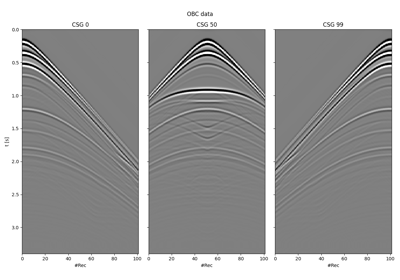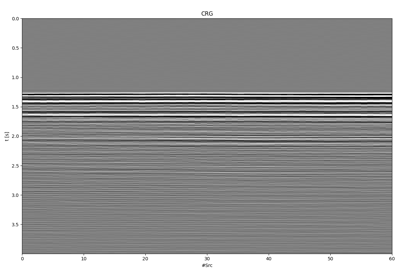# pylops.waveeqprocessing.BlendingContinuous#

class pylops.waveeqprocessing.BlendingContinuous(nt, nr, ns, dt, times, dtype='float64', name='B')[source]#

Continuous blending operator

Blend seismic shot gathers in continuous mode based on pre-defined sequence of firing times. The size of input model vector must be $$n_s \times n_r \times n_t$$, whilst the size of the data vector is $$n_r \times n_{t,tot}$$.

Parameters
ntint

Number of time samples

nrint

nsint

Number of sources

dtfloat

Time sampling in seconds

timesnp.ndarray

Absolute ignition times for each source

nprocint, optional

Number of processors used when applying operator

dtypestr, optional

Operator dtype

namestr, optional

Name of operator (to be used by pylops.utils.describe.describe)

Notes

Simultaneous shooting or blending is the process of acquiring seismic data firing consecutive sources at short time intervals (shorter than the time requires for all significant waves to come back from the Earth interior).

Continuous blending refers to an acquisition scenario where a source towed behind a single vessel is fired at irregular time intervals (times) to create a continuous recording whose modelling operator is

$\Phi = [\Phi_1, \Phi_2, ..., \Phi_N]$

where each $$\Phi_i$$ operator applies a time-shift equal to the absolute ignition time provided in the variable times.

Methods

 __init__(nt, nr, ns, dt, times[, dtype, name]) adjoint() apply_columns(cols) Apply subset of columns of operator cond([uselobpcg]) Condition number of linear operator. conj() Complex conjugate operator div(y[, niter, densesolver]) Solve the linear problem $$\mathbf{y}=\mathbf{A}\mathbf{x}$$. dot(x) Matrix-matrix or matrix-vector multiplication. eigs([neigs, symmetric, niter, uselobpcg]) Most significant eigenvalues of linear operator. matmat(X) Matrix-matrix multiplication. matvec(x) Matrix-vector multiplication. reset_count() Reset counters rmatmat(X) Matrix-matrix multiplication. rmatvec(x) Adjoint matrix-vector multiplication. todense([backend]) Return dense matrix. toimag([forw, adj]) Imag operator toreal([forw, adj]) Real operator tosparse() Return sparse matrix. trace([neval, method, backend]) Trace of linear operator. transpose()

## Examples using pylops.waveeqprocessing.BlendingContinuous#Blending

Blending18. Deblending

18. Deblending